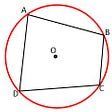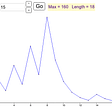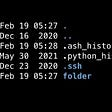# Line equations

point and a parallel line are given

r = a + lambda(b)

Cartesian: x = x1 — lambda(a)

x-x1/a = lambda

a is the point and lambda b is the parallel line: so the r (by triangle rule) is the sum of the two

two points

r = a + lambda(b-a)

x = x1 + lambda( x2-x1 )

here the parallel line ( lambda(b-a) ) is the subtraction of the two points given and just like before adding any vector of the two will give us r

x-x1/ x2-x1 = lambda

conventions to convert in Cartesian form

r = x, y,z

a = x1,y1, z1

b = x2 y2 z2

in the first case b was the parallel line, in case 2 we need to find the the parallel vector by b — a which is (x2-x1) which is the only thing different from the first case

quick note: because we are using parallel line in both case to determine line equation we will have lambda and the parallel line when ever we talk about line equation in either form

# plane

point and normal

r.ncap = d

lx + my + nz = d

point and (non normalized) normal vector

(r-a).N = 0

Cartesian form

A( x-x1 ) + B(y-y2) + C(z-z2) = 0

only in the first we will know the distance from the origin

--

--

--

## More from Arnav Zedian

Finding Magic.

Love podcasts or audiobooks? Learn on the go with our new app.Finding Magic.

## More from Medium## The 3n+1 problem — In simple terms## Near misses for disproving Fermat’s conjecture